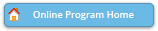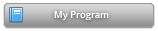Abstract Details

 Activity Number: 260 - SPEED: Topics in Bayesian Analysis Type: Contributed Date/Time: Monday, July 30, 2018 : 3:05 PM to 3:50 PM Sponsor: Section on Bayesian Statistical Science Abstract #332605 Title: A Theoretical Framework for Bayesian Nonparametric Regression: Orthonormal Random Series and Rates of Contraction Author(s): Fangzheng Xie* and Wei Jin and Yanxun Xu Companies: Johns Hopkins University and Johns Hopkins University and Johns Hopkins University Keywords: Bayesian nonparametric regression; integrated L2-distance; orthonormal random series; rate of contraction Abstract: We develop a unifying framework for Bayesian nonparametric regression to study the rates of contraction with respect to the integrated L2-distance without assuming the regression function space to be uniformly bounded. The framework is built upon orthonormal random series in a flexible manner. A general theorem for deriving rates of contraction for Bayesian nonparametric regression is provided under the proposed framework. As specific applications, we obtain the near-parametric rate of contraction for the squared-exponential Gaussian process when the true function is analytic, the adaptive rates of contraction for the sieve prior, and the adaptive-and-exact rates of contraction for the un-modified block prior when the true function is alpha-smooth. Extensions to wavelet series priors and fixed-design regression problems are also discussed.

Authors who are presenting talks have a * after their name.